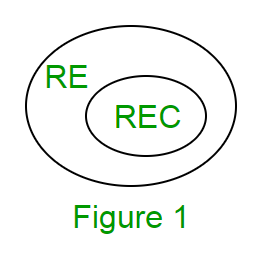# Recursive and Recursive Enumerable Languages in TOC

Recursive Enumerable (RE) or Type -0 Language

RE languages or type-0 languages are generated by type-0 grammars. An RE language can be accepted or recognized by Turing machine which means it will enter into final state for the strings of language and may or may not enter into rejecting state for the strings which are not part of the language. It means TM can loop forever for the strings which are not a part of the language. RE languages are also called as Turing recognizable languages.

Recursive Language (REC)

A recursive language (subset of RE) can be decided by Turing machine which means it will enter into final state for the strings of language and rejecting state for the strings which are not part of the language. e.g.; L= {anbncn|n>=1} is recursive because we can construct a turing machine which will move to final state if the string is of the form anbncn else move to non-final state. So the TM will always halt in this case. REC languages are also called as Turing decidable languages. The relationship between RE and REC languages can be shown in Figure 1.Closure Properties of Recursive Languages

• Union: If L1 and If L2 are two recursive languages, their union L1∪L2 will also be recursive because if TM halts for L1 and halts for L2, it will also halt for L1∪L2.
• Concatenation: If L1 and If L2 are two recursive languages, their concatenation L1.L2 will also be recursive. For Example:
```    L1= {anbncn|n>=0}
L2= {dmemfm|m>=0}
L3= L1.L2
= {anbncndm emfm|m>=0 and n>=0} is also recursive.
```

L1 says n no. of a’s followed by n no. of b’s followed by n no. of c’s. L2 says m no. of d’s followed by m no. of e’s followed by m no. of f’s. Their concatenation first matches no. of a’s, b’s and c’s and then matches no. of d’s, e’s and f’s. So it can be decided by TM.

• Kleene Closure: If L1is recursive, its kleene closure L1* will also be recursive. For Example:
```         L1= {anbncn|n>=0}
L1*= { anbncn||n>=0}* is also recursive.
```
• Intersection and complement: If L1 and If L2 are two recursive languages, their intersection L1 ∩ L2 will also be recursive. For Example:
```    L1= {anbncndm|n>=0 and m>=0}
L2= {anbncndn|n>=0 and m>=0}
L3=L1 ∩ L2
= { anbncndn |n>=0} will be recursive.
```

L1 says n no. of a’s followed by n no. of b’s followed by n no. of c’s and then any no. of d’s. L2 says any no. of a’s followed by n no. of b’s followed by n no. of c’s followed by n no. of d’s. Their intersection says n no. of a’s followed by n no. of b’s followed by n no. of c’s followed by n no. of d’s. So it can be decided by turing machine, hence recursive.
Similarly, complementof recursive language L1 which is ∑*-L1, will also be recursive.

Note: As opposed to REC languages, RE languages are not closed under complementon which means complement of RE language need not be RE.

GATE Questions

Question 1: Which of the following statements is/are FALSE?
1.For every non-deterministic TM, there exists an equivalent deterministic TM.
2.Turing recognizable languages are closed under union and complementation.
3.Turing decidable languages are closed under intersection and complementation.
4.Turing recognizable languages are closed under union and intersection.

A.1 and 4
B.1 and 3
C.2
D.3

Solution:

Statement 1 is true as we can convert every non-deterministic TM to deterministic TM.
Statement 2 is false as Turing recognizable languages (RE languages) are not closed under complementation.
Statement 3 is true as Turing decidable languages (REC languages) are closed under intersection and complementation.
Statement 4 is true as Turing recognizable languages (RE languages) are closed under union and intersection.

Question 2 : Let L be a language and L’ be its complement. Which one of the following is NOT a viable possibility?
A.Neither L nor L’ is RE.
B.One of L and L’ is RE but not recursive; the other is not RE.
C.Both L and L’ are RE but not recursive.
D.Both L and L’ are recursive.

Solution:

Option A is correct because if L is not RE, its complementation will not be RE. Option B is correct because if L is RE, L’ need not be RE or vice versa because RE languages are not closed under complementation.
Option C is false because if L is RE, L’ will not be RE. But if L is recursive, L’ will also be recursive and both will be RE as well because REC languages are subset of RE. As they have mentioned not to be REC, so option is false.
Option D is correct because if L is recursive L’ will also be recursive.

Question 3: Let L1 be a recursive language, and let L2 be a recursively enumerable but not a recursive language. Which one of the following is TRUE?

A.L1′ is recursive and L2′ is recursively enumerable
B.L1′ is recursive and L2′ is not recursively enumerable
C.L1′ and L2′ are recursively enumerable
D.L1′ is recursively enumerable and L2′ is recursive
Solution:

Option A is False as L2’ can’t be recursive enumerable (L2 is RE and RE are not closed under complementation).
Option B is correct as L1’ is REC (REC languages are closed under complementation) and L2’ is not recursive enumerable (RE languages are not closed under complementation).
Option C is False as L2’ can’t be recursive enumerable (L2 is RE and RE are not closed under complementation).
Option D is False as L2’ can’t be recursive enumerable (L2 is RE and RE languages are not closed under complementation). As REC languages are subset of RE, L2’ can’t be REC as well.